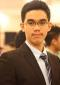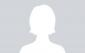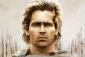# Discussion on: Application of Maxima and Minima

4 posts / 0 new
Klahrinz Willia...Discussion on: Application of Maxima and Minima

Following is a discussion on the Reviewer item titled: Application of Maxima and Minima. Feel free to add your own comments!

Klahrinz Willia...Okay, so I got really confused at part 1 of the problems.

There are some problems that differentiate likes this:
Problem 8
Let x and y the numbers
x+y=a
1+y′=0
y=−1

and there are some that do this:
Problem 6
Let x and y = the numbers
x+y=a
x=a−y

how do I know which one to use and when?

Richele TanI'm also confused on that, since I'm using a lot of book reference they don't use derivatives for the first equation. They only equate it to zero (derive) on the equation to be maximized/ minimized.

AlexanderI think it is about implicit and explicit type o differentiation. The first is differentiation under several variables, the other one is differentiation under single variable. When to use which, depends on the equation. Implicit differentiation will simplify complex process in explicit. There are times that it is is the other way around.

• Mathematics inside the configured delimiters is rendered by MathJax. The default math delimiters are $$...$$ and $...$ for displayed mathematics, and $...$ and $...$ for in-line mathematics.